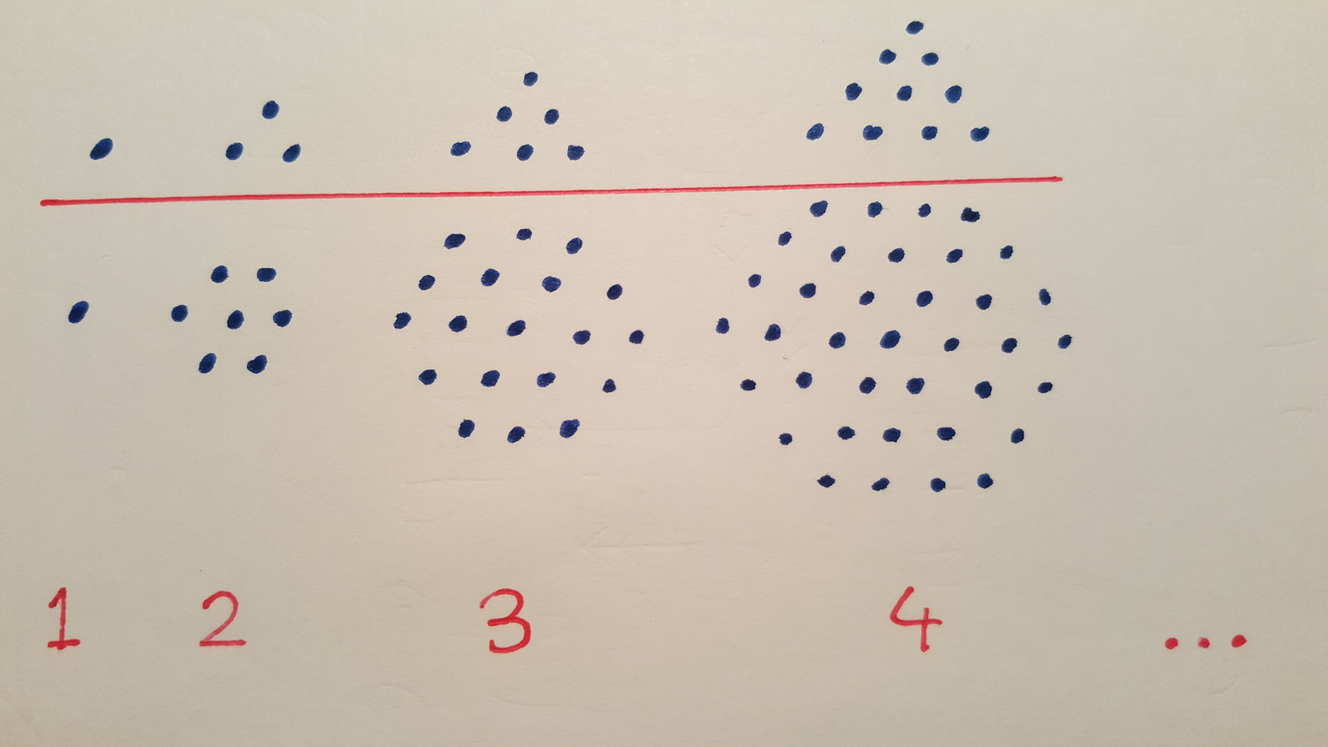# Hexagonal Numbers

Probability Level 2In the diagram above, we see a series of triangles and hexagons. How many dots are contained in the $20^\text{th}$ hexagon?

×